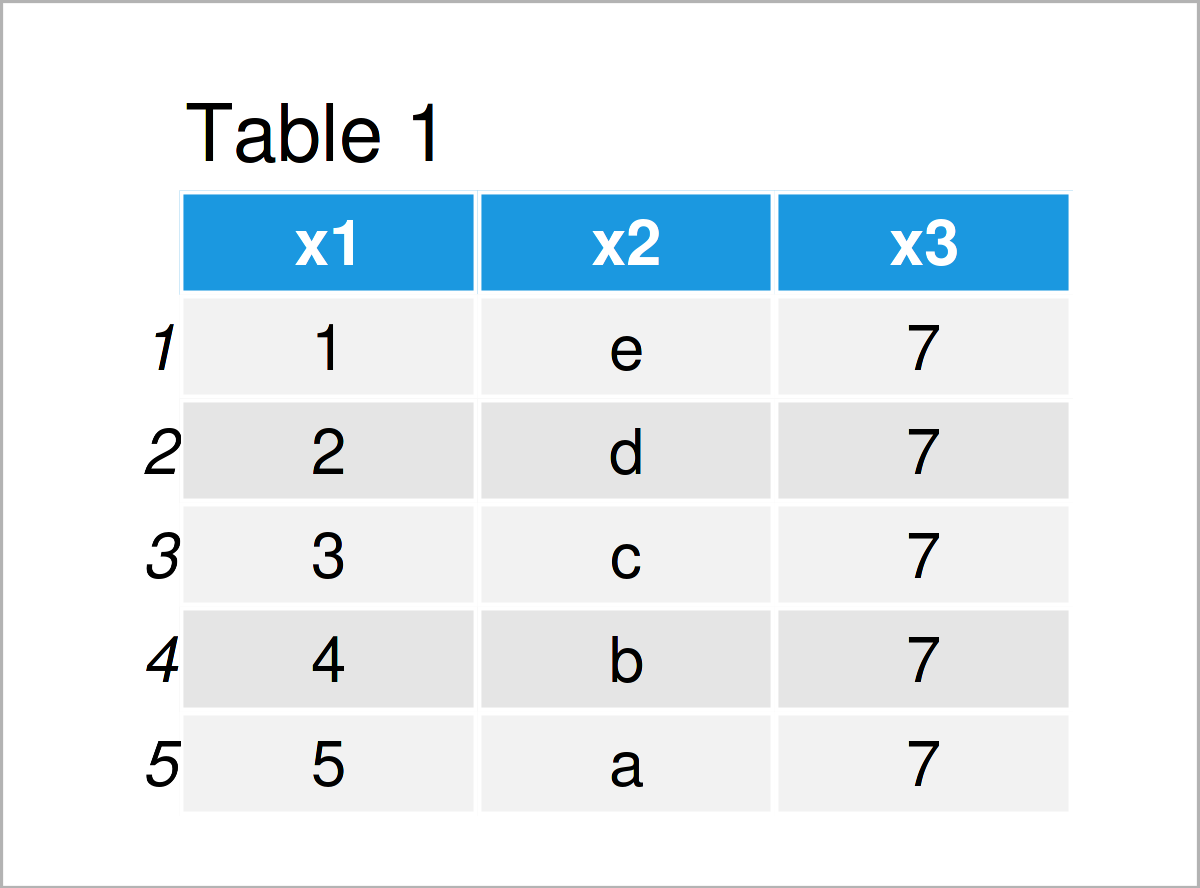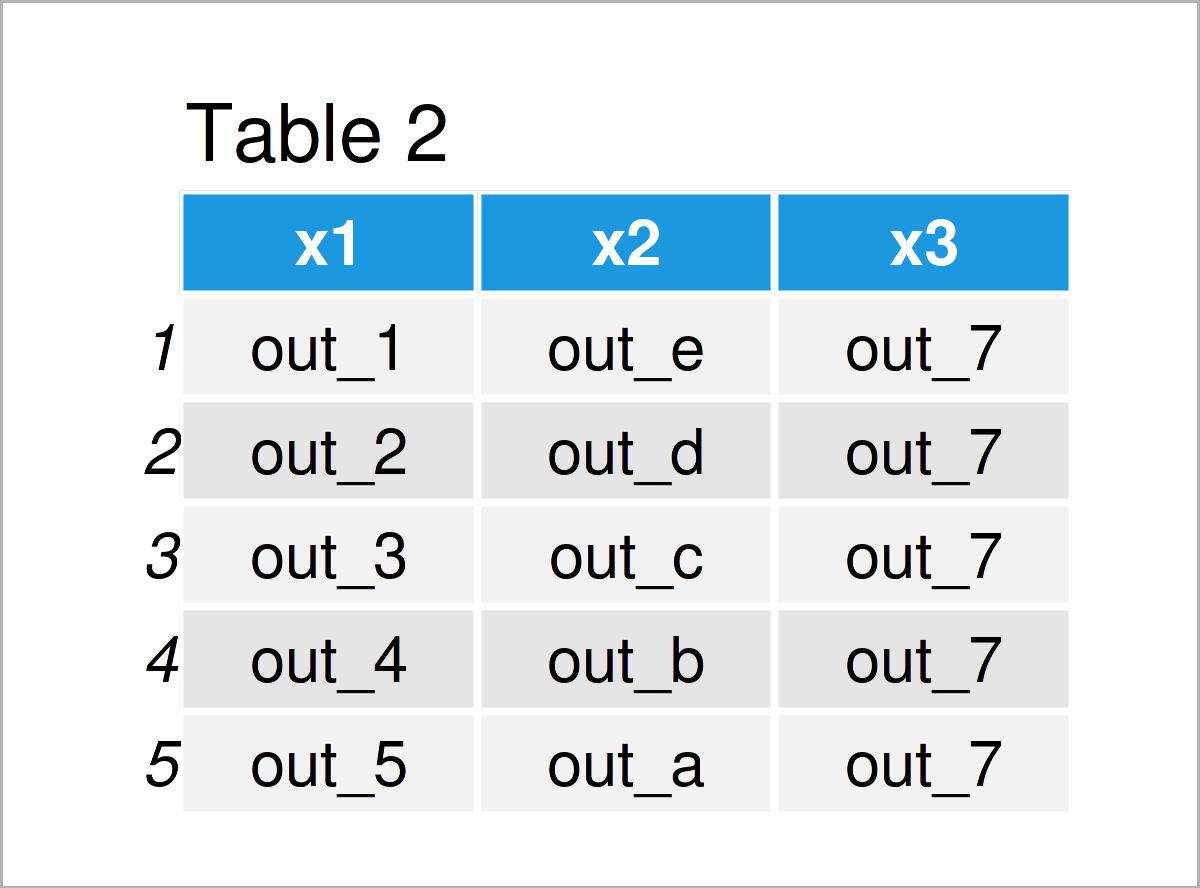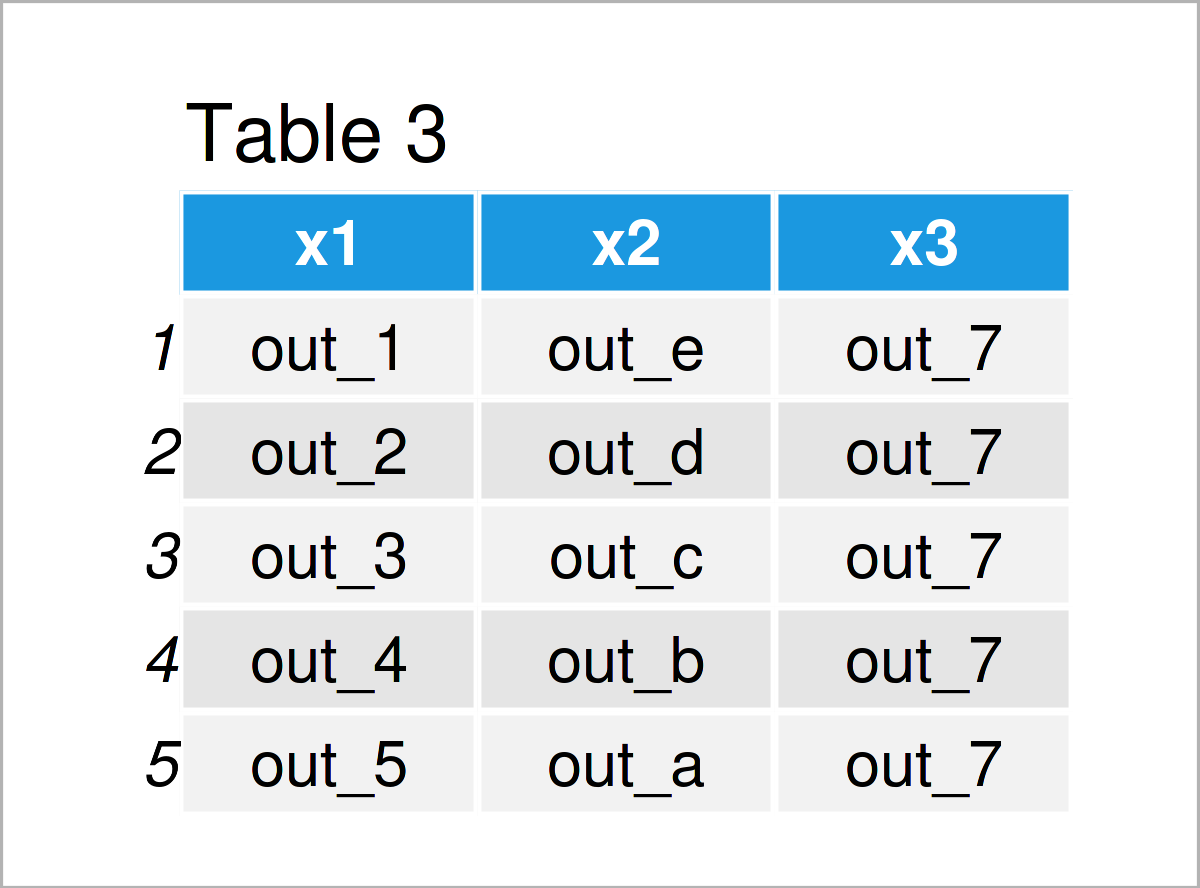# Apply Function to Each Cell of Data Frame in R (2 Examples)

In this article you’ll learn how to apply a user-defined function to each data frame element in the R programming language.

The tutorial consists of the following information:

Let’s dive into it:

## Construction of Exemplifying Data

We’ll use the following data as a basement for this R tutorial:

```data <- data.frame(x1 = 1:5,                # Create example data
x2 = letters[5:1],
x3 = 7)
data                                        # Print example data```Table 1 shows that the example data consists of five rows and three columns.

Next, let’s create a user-defined function:

```my_fun <- function(x) {                     # Create user-defined function
paste0("out_", x)
}```

The previous R code has created a new manually defined function called my_fun. We can apply this function to a single data cell as shown below:

```my_fun(data\$x1)                          # Apply function to one value
#  "out_3"```

As you can see, our function adds the prefix “out_” to it’s input.

Let’s apply this function to all elements in our example data frame!

## Example 1: Apply User-Defined Function to Each Element of Data Frame Using lapply() Function

Example 1 shows how to apply a user-defined function to every cell of a data set using Base R.

For this task, we can use the lapply function as shown below.

Note that we are specifying [] after the name of the data frame. This keeps the structure of our data. If we wouldn’t use this operator, the lapply function would return a list object.

```data_new1 <- data                           # Duplicate data frame
data_new1[] <- lapply(data_new1, my_fun)    # Apply function to each element
data_new1                                   # Print updated data frame```As shown in Table 2, the previous R syntax has created a new data frame called data_new1 where each cell contains the prefix “out_”.

## Example 2: Apply User-Defined Function to Each Element of Data Frame Using mutate_all() Function of dplyr Package

This example illustrates how to use the dplyr package instead of Base R to apply a function to each data cell.

We first need to install and load the dplyr package, in order to use the corresponding functions:

```install.packages("dplyr")                   # Install & load dplyr
library("dplyr")```

Next, we can apply the mutate_all function to use our function for each data cell:

```data_new2 <- data %>%                       # Apply function to each element
mutate_all(my_fun)
data_new2                                   # Print updated data frame```Table 3 shows the output of the previous syntax: The same data frame that we have already created in Example 2.

## Video, Further Resources & Summary

Do you want to know more about the application of a user-defined function to each data frame element? Then you might want to have a look at the following video on my YouTube channel. In the video, I explain the R programming syntax of this page.

Besides the video, you might read the other articles on this website:

Summary: In this R tutorial you have learned how to apply a user-defined function to each data frame cell. Please tell me about it in the comments section below, in case you have any further comments and/or questions.

Subscribe to the Statistics Globe Newsletter